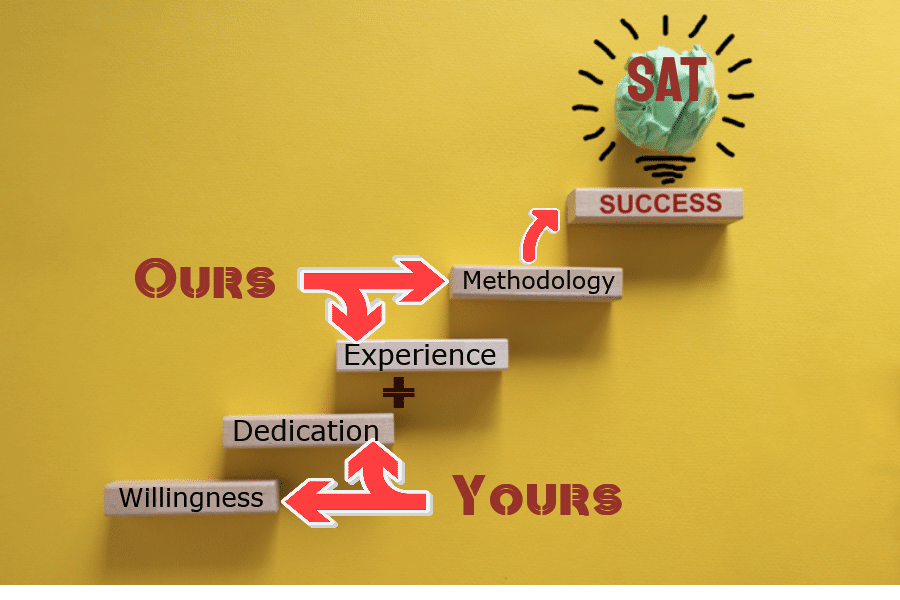## Why Scholars Point!

Our Vedic Math Techniques:

• Will increase your speed and accuracy
• Remove Silly Mistakes

## We offer:

• One to One SAT Preparation classes
• Small groups (Max intake 6 students) classes
• Customized Classes as per ones need
• Weekdays / weekend classes

## SAT Exam Highlights:

Test Duration: 02 Hours
Mode of Test: Written-based examination (Digital in 2023)
Age Limit : No age limit ( but maximum aspirant are between 17 to 19 years)
Maximum Attempts : No Limit
Eligibility criteria : No criteria as such, but generally taken by students who are in high school
Official Website : //satsuite.collegeboard.org/satt
Acceptance: US, Canada, also accepted in UK and Australia
Organised by: The College Board

## Test Structure of Math Test for SAT Exam:

Total Math Questions: 58
Total Time : 80 Minutes

Math Test– No Calculator
Time: 25 Minutes
Questions: 20

Math Test–Calculator
Time: 55 Minutes
Questions: 38

Marking : No Negative Marking
Type of Questions : Maximum Questions are MCQs

## SAT Exam Syllabus for Maths

### Heart of Algebra

• Create, solve, or interpret a linear expression or equation in 1 variable.
• Create, solve, or interpret linear inequalities in 1 variable.
• Build a linear function that models a linear relationship between 2 quantities.
• Create, solve, and interpret systems of linear inequalities in 2 variables.
• Create, solve, and interpret systems of 2 linear equations in 2 variables.
• Algebraically solve linear equations (or inequalities) in 1 variable.
• Algebraically solve systems of 2 linear equations in 2 variables.
• Interpret the variables and constants in expressions for linear functions.
• Understand connections between algebraic and graphical representations.

### Problem Solving and Data Analysis:

• Use ratios, rates, proportional relationships, and scale drawings to solve single- and multistep problems.
• Solve single- and multistep problems involving percentages.
• Solve single- and multistep problems involving measurement quantities, units, and unit conversion.
• Use scatterplot, linear, quadratic, or exponential models to describe how the variables are related.
• Use the relationship between 2 variables to investigate key features of the graph.
• Compare linear growth with exponential growth.
• Use 2-way tables to summarize categorical data and relative frequencies and calculate conditional probability.
• Make inferences about population parameters based on sample data.
• Use statistics to investigate measures of center of data. Analyze shape, center, and spread.
• Evaluate reports to make inferences, justify conclusions, and determine appropriateness of data collection methods.
• The reports may consist of tables, graphs, or text summaries.

• Create a quadratic or exponential function or equation that models a context.
• Determine the most suitable form of an expression or equation to reveal a particular trait, given a context.
• Create equivalent expressions involving rational exponents and radicals, which includes simplifying or rewriting in other forms.
• Create an equivalent form of an algebraic expression by using structure and fluency with operations.
• Solve a quadratic equation having rational coefficients. The equation can be presented in a wide range of forms to reward attending to algebraic structure and can require manipulation to solve.
• Add, subtract, and multiply polynomial expressions. Simplify the result. The expressions will have rational coefficients.
• Solve an equation in 1 variable that contains radicals or contains the variable in the denominator of a fraction.
• Solve a system of 1 linear equation and 1 quadratic equation.
• Rewrite simple rational expressions.
• Interpret parts of nonlinear expressions in terms of their context.
• Understand the relationship between zeros and factors of polynomials. Use that knowledge to sketch graphs.
• Understand a nonlinear relationship between 2 variables by making connections between their algebraic and graphical representations.
• Use function notation, and interpret statements using function notation.
• Use structure to isolate or identify a quantity of interest in an expression or isolate a quantity of interest in an equation.